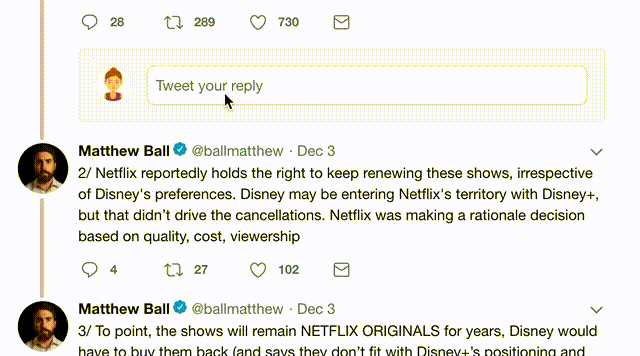, 17 tweets, 5 min read
A small thread about (part of) the solution to this problem! (1/n)For now, let's just think about computing the fundamental group, π_1(X). First things first: what exactly are we working with?

For Topology questions, often a good start is to ask yourself "What's the picture?"
(2/n)
Here's a depiction: we're starting with two 2-spheres (in equations, just subsets of Euclidean space of the form (x-a)^2 + (y-b)^2 = r^2), here A and B.

Both have an "equator", i.e. a distinguished embedded copy of a circle S^1, say all of the points with y coordinate 0.
(3/n)We're also given a map f from one equator to the other, which is 2-sheeted, which says every point in the image should pull back to 2 points in the preimage -- i.e., it wraps one circle around the other twice. We want to now "glue" the two spaces together along this map.
(4/n)
You construct this space by taking a disjoint union, then taking a quotient by the equivalence relation z ~ f(z).

If the map were the identity, we would get something homeomorphic to this space: one sphere inside another, with the equators "zipped" together in orange.

(5/n)Since our map isn't trivial, this is just a cartoon for now, but we can try to use it to reason about how the formal proof will go.

Whenever you have these "adjunction" spaces given by gluing, using the Seifert van-Kampen theorem to compute π_1 is usually a good bet.

(6/n)
This theorem says that if we can decompose our space X = A∪B into two "nice" (path-connected and nonempty) pieces that we understand *and* whose intersection we understand, we can assemble this information to get π_1 X.

So first up is looking for this decomposition.
(7/n)
Recall that we had this picture for X, two S^2s glued along an orange equator. What we do now is a common technique: we'll let A be (say) all of the green sphere, and we'll extend it a little bit beyond the glued region into the blue sphere.

(8/n)So one of our pieces will be this Ã, i.e. all of A and a small neighborhood of the equator in B, all sitting inside of the glued space X. This ends up looking like a usual sphere with a small rectangular band sewed along its equator.

(9/n)And (importantly!) in Ã, the blue bit is homeomorphic to an interval, which is contractible. So we can pull all of the blue back onto the equator without changing its homotopy type.

So Ã deformation retracts onto A = S^2, and we understand that pretty well.

(10/n)So now we just play the same game with the other sphere: we'll take our other piece to be all of B, extended a tiny bit beyond the gluing, into A. The picture's a bit different, but the conclusion is the same, i.e. it deformation retracts onto B=S^2Finally, we want to understand their intersection: we know they intersect along the equator, because each contains the equators that were glued together.

And each extends a bit into the other, so we get the equator along with its neighborhoods in A and B.
(12/n)And we can deformation retract this, now along both the green and blue lines, which shrinks it all onto the equator. So we get a homotopy equivalence between the full intersection shown and the orange S^1.

(13/n)
But the claim is that now the hard work is done! Algebraic Topology 1 tells us that π_1 S^1 = ℤ and π_1 S^2 = 0, and Seifert-van Kampen tells us precisely how they combine: by taking a certain pushout of the groups appearing, i.e. an "amalgamated product".

(14/n)
Here's the catch: we are taking an amalgamated product of trivial groups, and so we can immediately conclude that the result is trivial too! So we got pretty lucky: if both pieces had nontrivial π_1, we would need to compute this amalgamation using the double cover map.

(15/n)So a few challenges!

1. How can you modify the original problem so we get nontrivial π_1 in the pieces?

2. Is this decomposition enough to use Mayer-Vietoris to compute the homology H_*(X)?

3. Where did the dimension matter? Does this work for S^{n-1} in S^n?

(16/16) ✌️😁
Missing some Tweet in this thread? You can try to force a refresh.

Keep Current with D. Zack Garza

Stay in touch and get notified when new unrolls are available from this author!

This Thread may be Removed Anytime!Twitter may remove this content at anytime, convert it as a PDF, save and print for later use!

# Try unrolling a thread yourself!2) Go to a Twitter thread (series of Tweets by the same owner) and mention us with a keyword "unroll" `@threadreaderapp unroll`